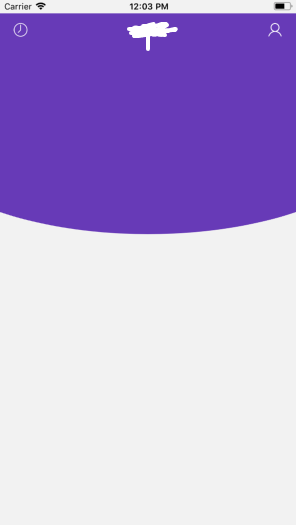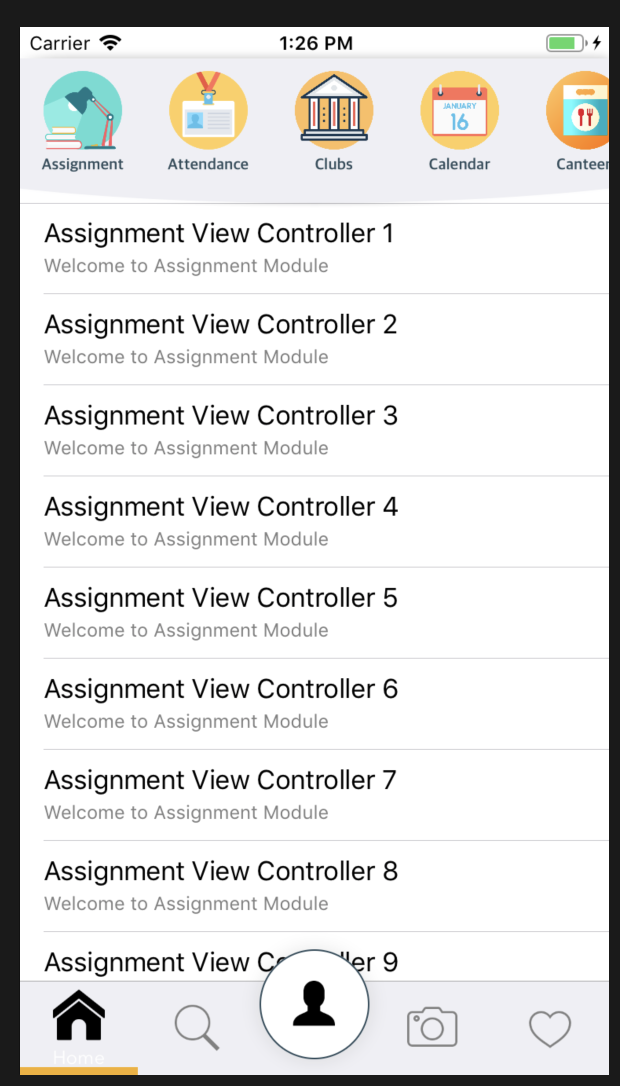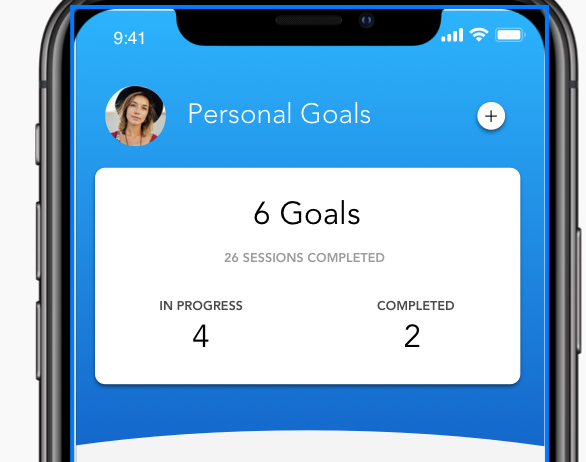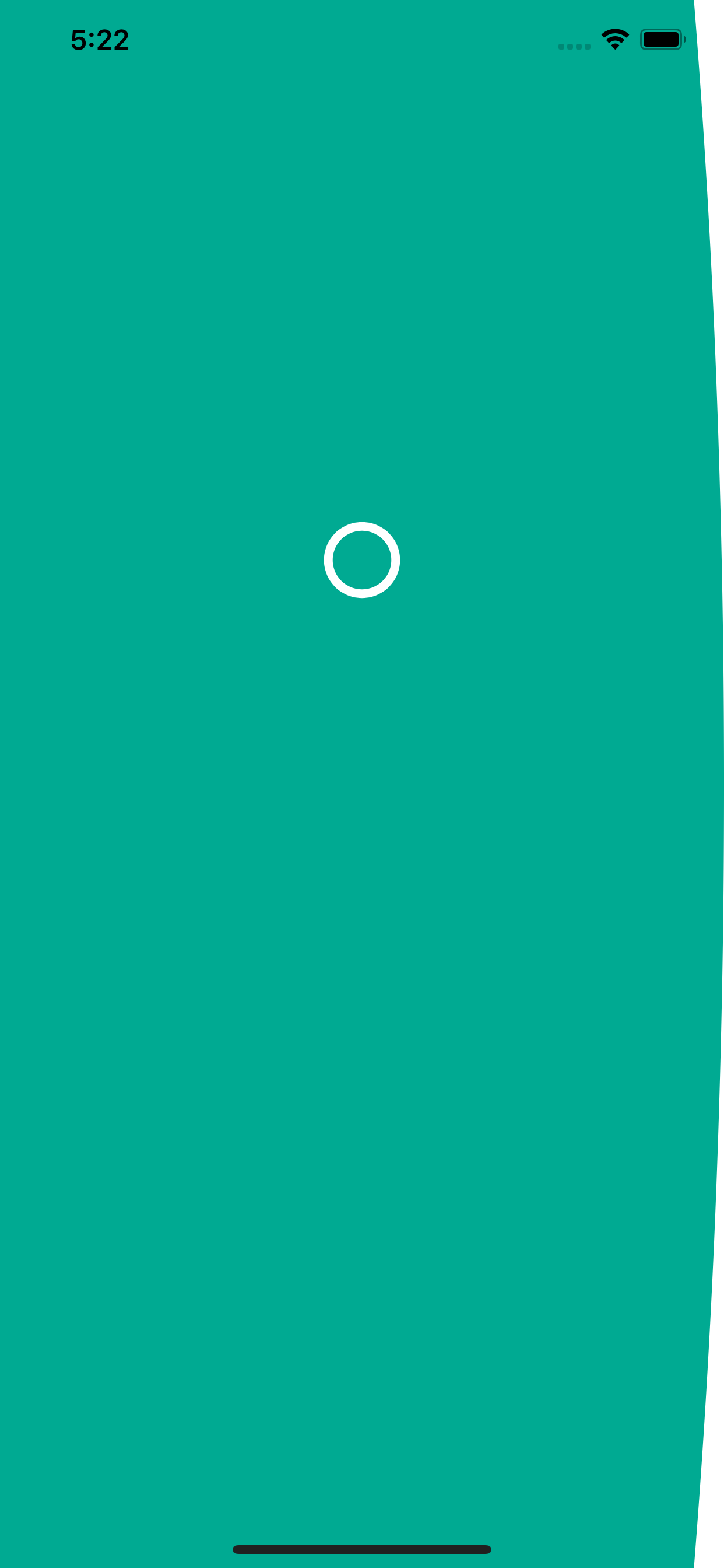{{ message }}

Instantly share code, notes, and snippets.

# anitaa1990/CurvedUIView.swift

Last active Apr 12, 2021
A Swift extension of UIView to display a curved view
 import UIKit extension UIView { /* Usage Example * bgView.addBottomRoundedEdge(desiredCurve: 1.5) */ func addBottomRoundedEdge(desiredCurve: CGFloat?) { let offset: CGFloat = self.frame.width / desiredCurve! let bounds: CGRect = self.bounds let rectBounds: CGRect = CGRect(x: bounds.origin.x, y: bounds.origin.y, width: bounds.size.width, height: bounds.size.height / 2) let rectPath: UIBezierPath = UIBezierPath(rect: rectBounds) let ovalBounds: CGRect = CGRect(x: bounds.origin.x - offset / 2, y: bounds.origin.y, width: bounds.size.width + offset, height: bounds.size.height) let ovalPath: UIBezierPath = UIBezierPath(ovalIn: ovalBounds) rectPath.append(ovalPath) // Create the shape layer and set its path let maskLayer: CAShapeLayer = CAShapeLayer() maskLayer.frame = bounds maskLayer.path = rectPath.cgPath // Set the newly created shape layer as the mask for the view's layer self.layer.mask = maskLayer } }

###anitaa1990 commented Mar 31, 2018 • edited

 ``````/* Usage Example */ bgView.addBottomRoundedEdge(desiredCurve: 1.5) ``````###RishabhSRS commented Sep 20, 2018

 hey , thanks for this code ! can you help me out for getting the shadow for that curve as well bit confused !###RishabhSRS commented Sep 20, 2018 • edited

 getting that shadow only for the curve edge as you can see modified your code little bit but was not able to achieve `func addBottomRoundedEdge(desiredCurve: CGFloat?,borderColor: CGColor) { let offset: CGFloat = self.frame.width / desiredCurve! let bounds: CGRect = self.bounds `````` let rectBounds: CGRect = CGRect(x: bounds.origin.x, y: bounds.origin.y, width: bounds.size.width, height: bounds.size.height / 2) let rectPath: UIBezierPath = UIBezierPath(rect: rectBounds) let ovalBounds: CGRect = CGRect(x: bounds.origin.x - offset / 2, y: bounds.origin.y, width: bounds.size.width + offset, height: bounds.size.height) let ovalPath: UIBezierPath = UIBezierPath(ovalIn: ovalBounds) rectPath.append(ovalPath) let maskLayer: CAShapeLayer = CAShapeLayer() maskLayer.frame = bounds maskLayer.path = rectPath.cgPath maskLayer.shadowColor = borderColor maskLayer.shadowOpacity = 10.0 self.layer.mask = maskLayer self.layer.borderWidth = 0 self.layer.borderColor = borderColor }` ``````

###shubhjagani commented Jan 13, 2019

 Do you know how to inverse the curvature?###texyz commented Jan 14, 2020

 Please how can i inverse the curvature?

###ajkerfaisal commented Feb 14, 2020

 Did anyone figure out shadow only on the curve edge?

###yogiguruprasad commented Apr 28, 2020

 Do you know how to inverse the curvature?func addTopRoundedCornerToView(targetView:UIView?, desiredCurve:CGFloat?) { let offset:CGFloat = targetView!.frame.width/desiredCurve! let bounds: CGRect = targetView!.bounds `````` let rectBounds: CGRect = CGRect(x: bounds.origin.x, y: bounds.origin.y+bounds.size.height / 2, width: bounds.size.width, height: bounds.size.height / 2) let rectPath: UIBezierPath = UIBezierPath(rect: rectBounds) let ovalBounds: CGRect = CGRect(x: bounds.origin.x - offset / 2, y: bounds.origin.y, width: bounds.size.width + offset, height: bounds.size.height) let ovalPath: UIBezierPath = UIBezierPath(ovalIn: ovalBounds) rectPath.append(ovalPath) // Create the shape layer and set its path let maskLayer: CAShapeLayer = CAShapeLayer() maskLayer.frame = bounds maskLayer.path = rectPath.cgPath // Set the newly created shape layer as the mask for the view's layer targetView!.layer.mask = maskLayer } ``````

###Alkalouti commented May 6, 2020

 great job How I can make it from the left side I tried this code but it's appeared on the right `func addLeftRoundedEdge() { let offset: CGFloat = (self.frame.height * 1.5) let bounds: CGRect = self.bounds `````` let rectBounds: CGRect = CGRect(x: bounds.origin.x, y: bounds.origin.y, width: bounds.size.width/2 , height: bounds.size.height) let rectPath: UIBezierPath = UIBezierPath(rect: rectBounds) let ovalBounds: CGRect = CGRect(x: bounds.origin.x , y: bounds.origin.y - offset / 2, width: bounds.size.width , height: bounds.size.height + offset) let ovalPath: UIBezierPath = UIBezierPath(ovalIn: ovalBounds) rectPath.append(ovalPath) let maskLayer: CAShapeLayer = CAShapeLayer() maskLayer.frame = bounds maskLayer.path = rectPath.cgPath self.layer.mask = maskLayer }` ``````###chris--young commented May 27, 2020

 for people trying to add a shadow to this, i was able to do it by using a second view ``````class CurvedWithShadowView: UIView { private let shadowView = UIView() private let curvedView = UIView() init() { super.init(frame: .zero) shadowView.backgroundColor = .none shadowView.configureForAutoLayout() curvedView.backgroundColor = .white curvedView.configureForAutoLayout() addSubview(shadowView) addSubview(curvedView) configureForAutoLayout() setNeedsUpdateConstraints() } required init?(coder: NSCoder) { fatalError("init(coder:) has not been implemented") } // this is using "PureLayout" you could do the same with plain auto layout too override func updateConstraints() { shadowView.autoPinEdge(toSuperviewEdge: .top) shadowView.autoPinEdge(toSuperviewEdge: .bottom) shadowView.autoPinEdge(toSuperviewEdge: .left) shadowView.autoPinEdge(toSuperviewEdge: .right) curvedView.autoPinEdge(toSuperviewEdge: .top) curvedView.autoPinEdge(toSuperviewEdge: .bottom) curvedView.autoPinEdge(toSuperviewEdge: .left) curvedView.autoPinEdge(toSuperviewEdge: .right) super.updateConstraints() } override func layoutSubviews() { let offset: CGFloat = frame.width / 0.5 let mask: CAShapeLayer = CAShapeLayer() let rectBounds: CGRect = CGRect(x: bounds.origin.x, y: bounds.origin.y + bounds.size.height / 2, width: bounds.size.width, height: bounds.size.height / 2) let rectPath: UIBezierPath = UIBezierPath(rect: rectBounds) let ovalBounds: CGRect = CGRect(x: bounds.origin.x - offset / 2, y: bounds.origin.y, width: bounds.size.width + offset, height: bounds.size.height) let ovalPath: UIBezierPath = UIBezierPath(ovalIn: ovalBounds) rectPath.append(ovalPath) mask.frame = bounds mask.path = rectPath.cgPath curvedView.layer.mask = mask shadowView.layer.shadowPath = rectPath.cgPath shadowView.layer.shadowColor = UIColor.black.cgColor shadowView.layer.shadowOpacity = 0.15 shadowView.layer.shadowOffset = .zero shadowView.layer.shadowRadius = 4.0 } } ``````